# Trying to understand space-time diagrams

• I
• Kashmir
A scalar product is a product of vectors that produces a scalar. It is not an inner product, which necessarily comes with the requirement of positive definiteness.f

#### Kashmir

I need your help. I've just begun learning about space time diagrams. Below is my doubt:

An event is shown as a point on a spacetime diagram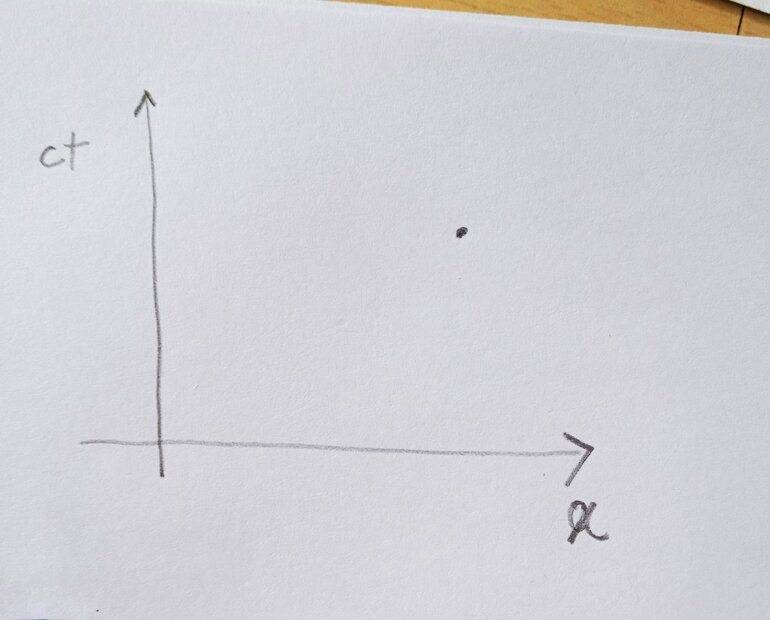Another frame has coordinates of space and time as:
##\begin{aligned} c t^{\prime} &=(\cosh \theta)(c t)-(\sinh \theta) x \\ x^{\prime} &=(-\sinh \theta)(c t)+(\cosh \theta) x \\ y^{\prime} &=y \\ z^{\prime} &=z \end{aligned}##

It's said that the spacetime diagram corresponding to the new frame could be drawn on the previous spacetime diagram as shown below: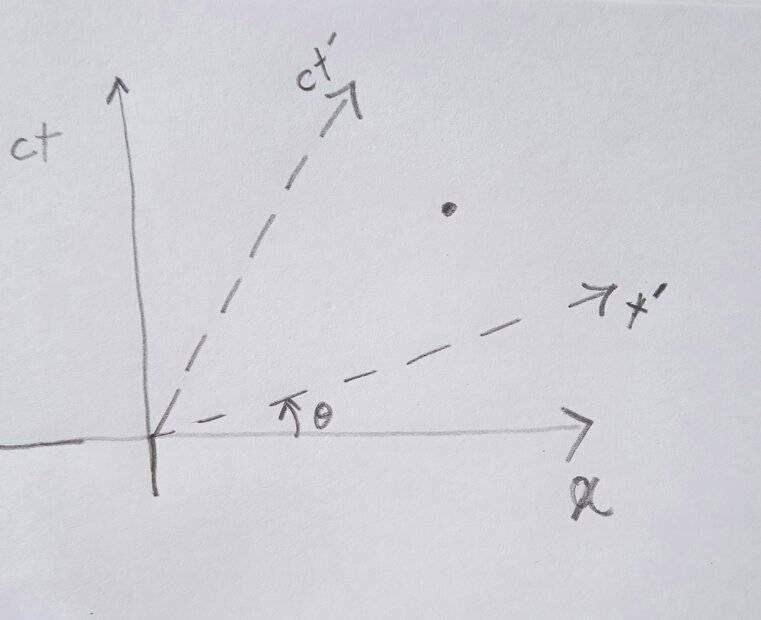Then is that the time coordinate of the event in the new frame can be found as shown below (red line is parallel to the ##x′## line, ##(a,b)## is the intersection point of red line with ##ct′##axis)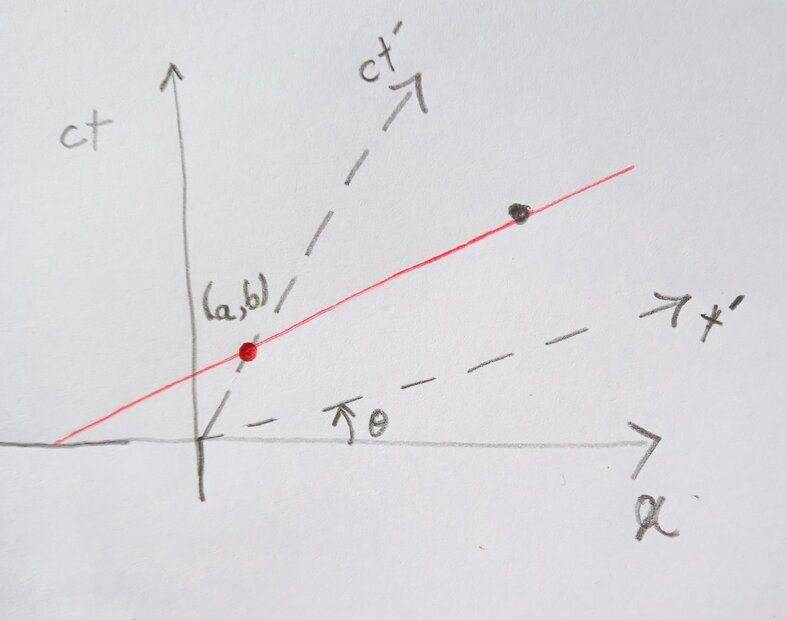Hence the new time coordinate is
##c t^{\prime}=\sqrt{a^2+b^2}##

? Is that correct?

No, it is ##\sqrt{a^2-b^2}##.

•topsquark, malawi_glenn and Kashmir
No, it is ##\sqrt{a^2-b^2}##.
Why? Can you please explain it?

Why? Can you please explain it?
Because Minkowski space geometry is not Euclidean. The Minkowski space version of the Pythagorean theorem is ##s^2 = (ct)^2 - x^2##.

•PhDeezNutz, Dale, topsquark and 3 others
One more proof of my caution with Minkowski diagrams in the introductory SR lecture! It must be made clear before (!) drawing the first Minkowski diagram that there is no Euclidean geometry to be applied in it. The entire geometry in the Minkowski plane is governed by the fundamental Lorentzian form with signature (1,1) not by that of the Euclidean fundamental form of signature (2,0). And only the Euclidean one is a scalar product, i.e., positive definite, and only this one induces a norm ("length", "metric").

•SolarisOne, SiennaTheGr8, Dale and 1 other person
And only the Euclidean one is a scalar product, i.e., positive definite, and only this one induces a norm ("length", "metric").
Well ... I think this is a question of terminology, technically it is a scalar product (a product of vectors that produces a scalar). It is, however, not an inner product, which necessarily comes with the requirement of positive definiteness. I have seen "scalar product" used in the more general sense as well as in the sense of being equivalent to an inner product (e.g., on Wikipedia (see second image) ... although that's not a solid reference of course).

•SiennaTheGr8 and Dale
Why? Can you please explain it?
The square of "distance" in Minkowski spacetime is not given by the Euclidean formula ##{(c\Delta t)^2+\Delta x^2}##, but by the Minkowski equivalent ##{(c\Delta t)^2-\Delta x^2}##. So the "length" of the line from the origin to ##(a,b)## (which is the time along it since this is a timelike interval) is ##\sqrt{a^2-b^2}##.

•SiennaTheGr8, Kashmir and topsquark
No, it is ##\sqrt{a^2-b^2}##.
Why? Can you please explain it?
It is because of this
Another frame has coordinates of space and time as:
##\begin{aligned} c t^{\prime} &=(\cosh \theta)(c t)-(\sinh \theta) x \\ x^{\prime} &=(-\sinh \theta)(c t)+(\cosh \theta) x \\ y^{\prime} &=y \\ z^{\prime} &=z \end{aligned}##
Notice that you used the hyperbolic ##\cosh## and ##\sinh## instead of the circular ##\cos## and ##\sin##. A circle is defined as ##r^2=x^2+y^2## but a hyperbola is defined as ##r^2=-t^2+x^2##. So when you are using hyperbolic "distances" you do hyperbolic rotations and vice versa.

This means that the distances you draw on the diagram are not directly the intervals in spacetime, and the angles that you draw on the diagram are not directly the rapidities in spacetime. On the paper distances are defined with circles and in spacetime intervals are defined with hyperbolas.

•SiennaTheGr8, PhDeezNutz, Kashmir and 4 others
Ok is this how we interpret the space-time diagram: Given a point as shown in the figure, we find the projections of that point on the axis, in our case we've oblique axes, then the coordinates are given as: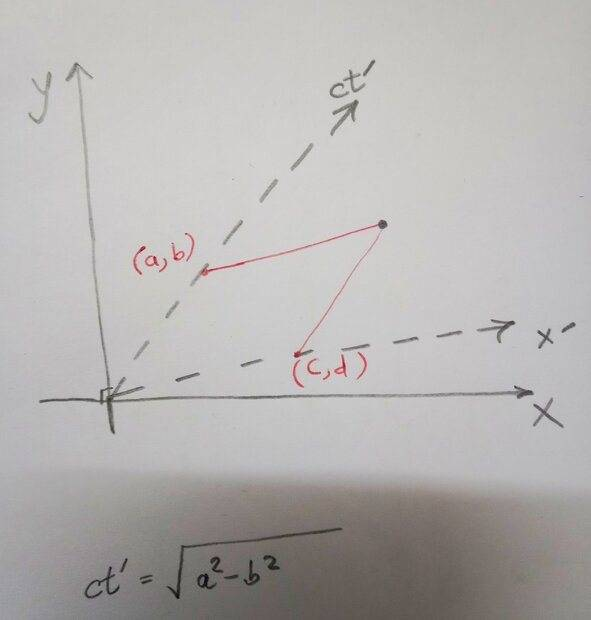##\begin{array}{l}
c t^{\prime}=\sqrt{a^2-b^2} \\
x^{\prime}=\sqrt{c^2-d^2}
\end{array}##

Now let's say I've another space-time diagram as shown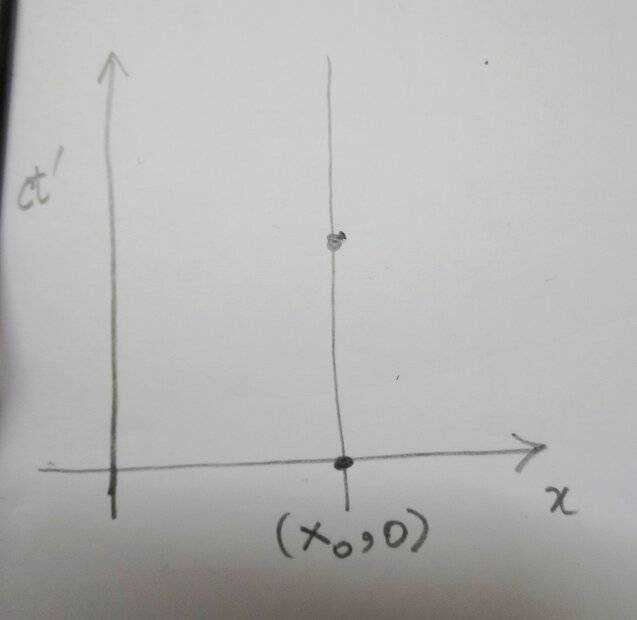In order to find the space coordinate of the point shouldn't I first find the projection on the ##x## axis and then write : ##x=\sqrt{x_0^2-0^2}##,which is simply how we used to do in euclidean geometry.

#### Attachments

Yes, that still is the same.

•topsquark
Yes, that still is the same.
Yes, but it makes the procedure consistent throughout.

Well ... I think this is a question of terminology, technically it is a scalar product (a product of vectors that produces a scalar). It is, however, not an inner product, which necessarily comes with the requirement of positive definiteness. I have seen "scalar product" used in the more general sense as well as in the sense of being equivalent to an inner product (e.g., on Wikipedia (see second image) ... although that's not a solid reference of course).
Ok, that seems to be once more something not uniquely defined. My math professors always insisted on the positive definiteness of a scalar product (bilinear form in real and sesquilinear form in complex vector spaces). The analogue for the more general case of an "indefinite scalar product" was named the "fundamental form" of a (real) vector space.

•topsquark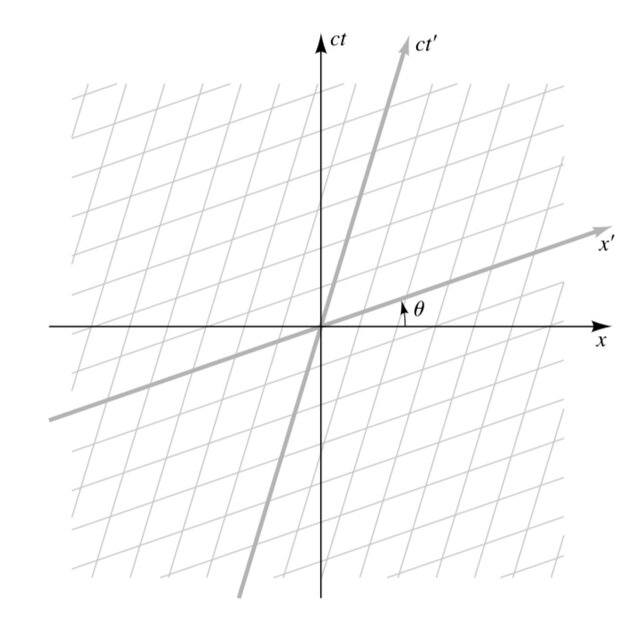I still don't understand how the angle between the two x and x'axes is ##\theta##?
Book writes
"FIGURE 4.13 A Lorentz boost as a change of coordinates on a spacetime diagram. The figure shows the grid of ##\left(c t^{\prime}, x^{\prime}\right)## coordinates defined by ##(4.18)## plotted on a ##(c t, x)## spacetime diagram. The ##\left(c t^{\prime}, x^{\prime}\right)## coordinates are not orthogonal to each other in the Euclidean geometry of the printed page. But they are orthogonal in the geometry of spacetime. (Recall the analogies between spacetime diagrams and maps discussed in Example 4.1.) The ##\left(c t^{\prime}, x^{\prime}\right)## axes have to be as orthogonal as the ##(c t, x)## axes because there is no physical distinction between one inertial frame and another. The orthogonality is explicitly verified in Example 5.2. The hyperbolic angle ##\theta## is a measure of the velocity between the two frames"

The transformation is given as

##\begin{aligned} c t^{\prime} &=(\cosh \theta)(c t)-(\sinh \theta) x \\ x^{\prime} &=(-\sinh \theta)(c t)+(\cosh \theta) x \\ y^{\prime} &=y \\ z^{\prime} &=z \end{aligned}##

To find the angle ##x'## axes makes with ##x## axes I use

##0=(\cosh \theta)(c t)-(\sinh \theta) x## to get its slope as ##Tanh(\theta)## and hence the angle is ##{Tan}^{-1}(Tanh(\theta))## but the book says the angle is ##\theta## ? What did I do wrong?

I still don't understand how the angle between the two x and x'axes is ##\theta##?
##\theta## is a hyperbolic angle, not a Euclidean angle. The angle ##\theta## you show in your diagram is a Euclidean angle, but that's not what ##\theta## is in your book.

##0=(\cosh \theta)(c t)-(\sinh \theta) x## to get its slope as ##Tanh(\theta)## and hence the angle is ##{Tan}^{-1}(Tanh(\theta))## but the book says the angle is ##\theta## ? What did I do wrong?
You treated ##\theta## as if it were a Euclidean angle; that's what using the inverse tangent function is doing.

••vanhees71, Kashmir and topsquark
To find the angle ##x'## axes makes with ##x## axes I use

##0=(\cosh \theta)(c t)-(\sinh \theta) x## to get its slope as ##Tanh(\theta)## and hence the angle is ##{Tan}^{-1}(Tanh(\theta))## but the book says the angle is ##\theta## ? What did I do wrong?
You used a circular inverse. It should be hyperbolic. I.e. ##{\tanh}^{-1}(\tanh(\theta))## and not ##{\tan}^{-1}(\tanh(\theta))##

This is hyperbolic geometry. Intervals are given by ##s^2=-t^2+x^2## and correspondingly all of the trig functions are hyperbolic as well.

•Kashmir, malawi_glenn and topsquark
One more proof of my caution with Minkowski diagrams in the introductory SR lecture! It must be made clear before (!) drawing the first Minkowski diagram that there is no Euclidean geometry to be applied in it. The entire geometry in the Minkowski plane is governed by the fundamental Lorentzian form with signature (1,1) not by that of the Euclidean fundamental form of signature (2,0). And only the Euclidean one is a scalar product, i.e., positive definite, and only this one induces a norm ("length", "metric").

Yes, be cautious.
Also be cautious with position-vs-time diagrams in PHY 101!
Here too,
there is no Euclidean geometry to be applied in it.
The entire geometry in the Galilean plane is governed by two fundamental degenerate forms with signature (1,0) and signature (0,1) not by that of the Euclidean fundamental form of signature (2,0). And only the Euclidean one is a scalar product, i.e., positive definite, and only this one induces a norm ("length", "metric“).

TL;DR
The geometry of any position-vs-time diagram (minkowski or Galilean) is not Euclidean!
(Be careful about drawing such diagrams in PHY 101!)

•Kashmir, topsquark and Dale
Walking through a field of Land mines!

Walking through a field of Land mines!

Don't be afraid. It's not that scary.
We're here to help.

••PhDeezNutz, Kashmir and topsquark
Don't be afraid. It's not that scary.
We're here to help.
Thank you
##\theta## is a hyperbolic angle, not a Euclidean angle. The angle ##\theta## you show in your diagram is a Euclidean angle, but that's not what ##\theta## is in your book.

You treated ##\theta## as if it were a Euclidean angle; that's what using the inverse tangent function is doing.

. So on the piece of paper the lines ##x, x'## have an angle of ##{\tan}^{-1}(\tanh{\theta})## but in the Minkowski space the angle is ##\theta##? Is that right?

View attachment 314507

I still don't understand how the angle between the two x and x'axes is ##\theta##?
Book writes
"FIGURE 4.13 A Lorentz boost as a change of coordinates on a spacetime diagram. The figure shows the grid of ##\left(c t^{\prime}, x^{\prime}\right)## coordinates defined by ##(4.18)## plotted on a ##(c t, x)## spacetime diagram. The ##\left(c t^{\prime}, x^{\prime}\right)## coordinates are not orthogonal to each other in the Euclidean geometry of the printed page. But they are orthogonal in the geometry of spacetime. (Recall the analogies between spacetime diagrams and maps discussed in Example 4.1.) The ##\left(c t^{\prime}, x^{\prime}\right)## axes have to be as orthogonal as the ##(c t, x)## axes because there is no physical distinction between one inertial frame and another. The orthogonality is explicitly verified in Example 5.2. The hyperbolic angle ##\theta## is a measure of the velocity between the two frames"

The transformation is given as

##\begin{aligned} c t^{\prime} &=(\cosh \theta)(c t)-(\sinh \theta) x \\ x^{\prime} &=(-\sinh \theta)(c t)+(\cosh \theta) x \\ y^{\prime} &=y \\ z^{\prime} &=z \end{aligned}##

To find the angle ##x'## axes makes with ##x## axes I use

##0=(\cosh \theta)(c t)-(\sinh \theta) x## to get its slope as ##Tanh(\theta)## and hence the angle is ##{Tan}^{-1}(Tanh(\theta))## but the book says the angle is ##\theta## ? What did I do wrong?
QED! Again someone confused by the fatal idea to call rapidities "angles" and then even draw them in a Euclidean way in the Minkowski plane, which has no Euclidean geometry by definition.

Rapidities are no angles! You cannot draw them as angles in a Minkowski diagram. You have to construct the hyperbolae and then they are areas!

•topsquark
QED! Again someone confused by the fatal idea to call rapidities "angles" and then even draw them in a Euclidean way in the Minkowski plane, which has no Euclidean geometry by definition.

Rapidities are no angles! You cannot draw them as angles in a Minkowski diagram. You have to construct the hyperbolae and then they are areas!
Hence the notation arsinh, artanh, etc. not arcsinh and arctanh as many seem to believe.

•••jtbell, topsquark, robphy and 1 other person
Ok everything I've so far said has been wrong.
However Is it true that the hyperbolic angles of all points on the line ##x'## are same and equal to ##\theta## ?
The hyperbolic angles defined by the sectors on the unit hyperbolas?

##0=(\cosh \theta)(c t)-(\sinh \theta) x## to get its slope as ##Tanh(\theta)## and hence the angle is ##{Tan}^{-1}(Tanh(\theta))## but the book says the angle is ##\theta## ? What did I do wrong?
You have to remember that the Minkowski plane is not the Euclidean plane, so the Minkowski diagram is an honest representation of the Minkowski plane but it is not an accurate representation. Just like a Mercator projection is an honest but not accurate representation of the surface of the Earth. You can't use Euclidean notions like length, angle, trigonometry. You have to use the Minkowski equivalents of interval, rapidity, and hyperbolic functions. They fit together in the same way - e.g. you take an inverse hyperbolic tangent where you would "intuitively" take an inverse tangent - which is why the answer is ##\theta=\tanh^{-1}\tanh\theta##, not ##\tan^{-1}\tanh\theta##

•topsquark and Kashmir
Ok everything I've so far said has been wrong.
However Is it true that the hyperbolic angles of all points on the line ##x'## are same and equal to ##\theta## ?
The hyperbolic angles defined by the sectors on the unit hyperbolas?
There are no angles! The meaning of the rapidity (here I use ##u## as a parameter) is as in the following picture: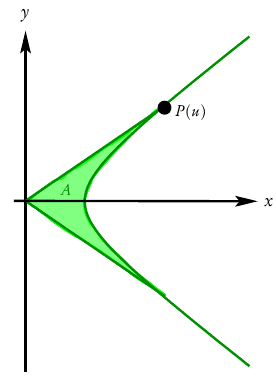The curve is the unit hyperbola
$$\vec{x}(u)=\begin{pmatrix} x(u) \\ y(u) \end{pmatrix}=\begin{pmatrix} \cosh u \\ \sinh u \end{pmatrix}, \quad u \in \mathbb{R}.$$
The green-shaded area can be parametrized as
$$\vec{x}(\lambda,u')=\lambda \vec{x}(u'), \quad \lambda \in [0,1], \quad u' \in [-u,u].$$
The area then is calculated using the Jacobi determinant
$$J=\partial_{\lambda} x \partial_u y - \partial_{\lambda} y \partial_u x=\lambda (\cosh^2 u'-\sinh^2 u')=\lambda,$$
i.e., the area is
$$A=\int_{-u}^u \mathrm{d} u' \int_0^1 \mathrm{d} \lambda \lambda = u,$$
i.e., ##u## is the area of the "hyperbolic segment" shaded in green.

That's why the inverse functions of the hyperbolic functions are called "area functions", i.e., arcosh, arsinh, artanh.

••Kashmir and topsquark
There are no angles!

In Euclidean geometry, one often hears the definition of angle as
##(\rm{angle})=\frac{\mbox{(arc-length along a circular arc)}}{\mbox{(radius of that circle)}}##.
This quantity is equal to the additive parameter ##\phi## used in a rotation,
and ##\tan\phi## is the slope and ##\cos\phi## is equal to the projection of a unit vector with respect to a reference axis.
The range ##0\leq \phi < 2\pi## covers the range of all possible directions.

In Minkowski spacetime geometry, one can declare the definition of M-angle as
##(\mbox{M-angle})=\frac{\mbox{(M-arc-length along a M-circular arc)}}{\mbox{(M-radius of the M-circle)}}##,
##(\mbox{rapidity})=\frac{\mbox{(spacelike-arc-length along a hyperbolic arc)}}{\mbox{(timelike-radius of the hyperbola)}}##
This quantity is equal to the additive parameter ##\theta## used in a Lorentz boost transformation,
and ##\tanh\theta## is the dimensionless-velocity and ##\cosh\theta## is equal to the time-dilation factor with respect to reference observer 4-velocity.
The range ##-\infty < \theta < \infty## covers the range of all possible timelike directions for a 4-velocity.

One can write an analogous description for Galilean spacetime geometry.
It turns out the Galilean analogue of the tangent function (Yaglom's ##\rm tang##)
satisfies ##{\rm tang\ }\eta=\eta##... so that, in Galilean relativity, velocities are additive [unlike in Minkowski spacetime geometry and "slopes" in Euclidean geometry.]

See page 7 of my old Spacetime Trigonometry poster:

Note: "rapidity" should be drawn using a hyperbolic arc, not a circular arc!

The above can also be expressed in terms of sector-areas* on a plane (as noted by @Orodruin and @vanhees71).
While the sectors are metric dependent (due to the choice of "circle"),
that "two areas are equal", or more generally, that "two given areas have a certain ratio"
is independent of metric in the plane...
since "comparing areas in the same plane" is an affine concept (not a metrical one).
It's akin to "comparing segments on the same line".

(*) Due to @Orodruin and @vanhees71, I have grown to embrace the "sector-area"- interpretation. I found a way to use it in my "rotated-graph paper" approach to describe rapidity and, analogously, proper-time for piecewise-constant-accelerated motions [forthcoming paper].
However, I have not abandoned the "arc-length of a unit circle"-interpretation because it works and because it makes contact with the more commonly used definition of angle.

Last edited:
•topsquark and vanhees71
There are no angles! The meaning of the rapidity (here I use ##u## as a parameter) is as in the following picture:

View attachment 314549
The curve is the unit hyperbola
$$\vec{x}(u)=\begin{pmatrix} x(u) \\ y(u) \end{pmatrix}=\begin{pmatrix} \cosh u \\ \sinh u \end{pmatrix}, \quad u \in \mathbb{R}.$$
The green-shaded area can be parametrized as
$$\vec{x}(\lambda,u')=\lambda \vec{x}(u'), \quad \lambda \in [0,1], \quad u' \in [-u,u].$$
The area then is calculated using the Jacobi determinant
$$J=\partial_{\lambda} x \partial_u y - \partial_{\lambda} y \partial_u x=\lambda (\cosh^2 u'-\sinh^2 u')=\lambda,$$
i.e., the area is
$$A=\int_{-u}^u \mathrm{d} u' \int_0^1 \mathrm{d} \lambda \lambda = u,$$
i.e., ##u## is the area of the "hyperbolic segment" shaded in green.

That's why the inverse functions of the hyperbolic functions are called "area functions", i.e., arcosh, arsinh, artanh.
So you defined rapidity ##\theta## as area that lies inside two lines that touch the unit hyperbola . If rapidity is ##\theta## then one of the intersection point of hyperbola with the line is ##(cosh(\theta), sinh(\theta)##.

Now getting back to our figure :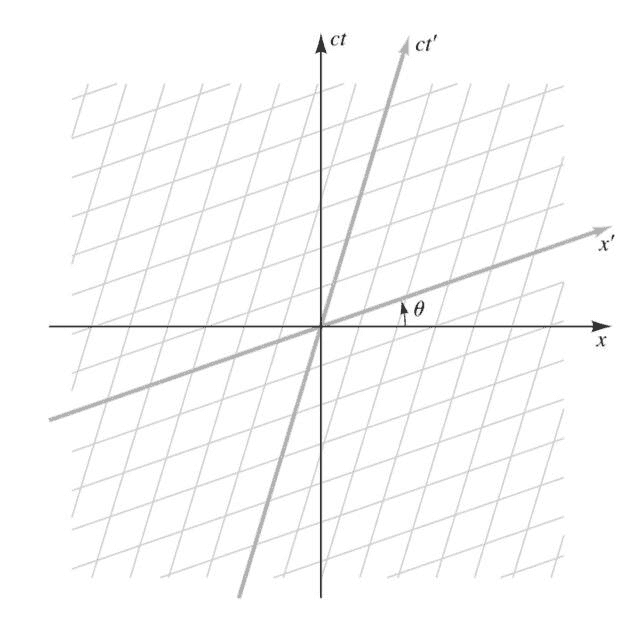The ##\theta## here is rapidity?

So you defined rapidity ##\theta## as area that lies inside two lines that touch the unit hyperbola . If rapidity is ##\theta## then one of the intersection point of hyperbola with the line is ##(cosh(\theta), sinh(\theta)##.

Now getting back to our figure :

View attachment 314558
The ##\theta## here is rapidity?
How are you defining ##\theta## on that diagram? For example, if we take ##v \to c##, what is the value of a) the rapidity; b) the ##\theta## shown on your diagram?

•topsquark
How are you defining ##\theta## on that diagram? For example, if we take ##v \to c##, what is the value of a) the rapidity; b) the ##\theta## shown on your diagram?
I am myself trying to understand that. My book gives me a transformation as:

##\begin{aligned} c t^{\prime} &=(\cosh \theta)(c t)-(\sinh \theta) x \\ x^{\prime} &=(-\sinh \theta)(c t)+(\cosh \theta) x \\ y^{\prime} &=y \\ z^{\prime} &=z \end{aligned}##

And gives me a plot :And then says that ##\theta## is the hyperbolic angle.

I've understood how we define hyperbolic angles or rather call them rapidity as shown by vanhees.

Now I want to link that concept of rapidity with the ##\theta## that's drawn on the book.

Now I want to link that concept of rapidity with the ##\theta## that's drawn on the book.
That's been explained several times already. Hyperbolic angles are a more abstract mathematical idea. They do not correspond to the internal angles on triangles drawn on a sheet of paper.

To answer my own question, as ##v \to c## the rapidity ##\theta \to \infty##. Whereas, the physical (Euclidean) angle shown on the diagram tends to ##\frac \pi 4##.

That means you have to reconcile the abstractness of Minkowski geometry with the physically Euclidean nature of any diagram you draw.

I would only add that algebra will never deceive you like this!

•vanhees71 and topsquark
"two given areas have a certain ratio"
is independent of metric in the plane...
Well, not generally. It just so happens that the area forms of 1+1-dimensional Minkowski space is equivalent to the area form of 2-dimensional Euclidean space. A different metric in the plane however will not have the same area form.

•vanhees71, topsquark and robphy
Well, not generally. It just so happens that the area forms of 1+1-dimensional Minkowski space is equivalent to the area form of 2-dimensional Euclidean space. A different metric in the plane however will not have the same area form.
I should clarify that I am restricting my discussion to the familiar (but not familiar-in-name) affine Cayley-Klein geometries (Euclidean, Galilean, and Minkowski) where there is an underlying vector-space structure, not a general 2-dimensional space.

•vanhees71 and topsquark
These diagrams may help you appreciate that "rapidity" can be thought of as an "angle",
when used properly. As with any tool, one must learn how to use it and how not-touse it.

Note the use of an arc of the "Minkowski circle"...
so we don't fall into the trap of thinking in terms of Euclidean geometry, as @vanhees71 warns us about.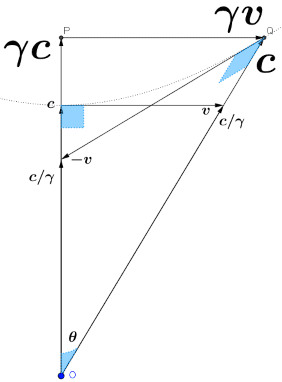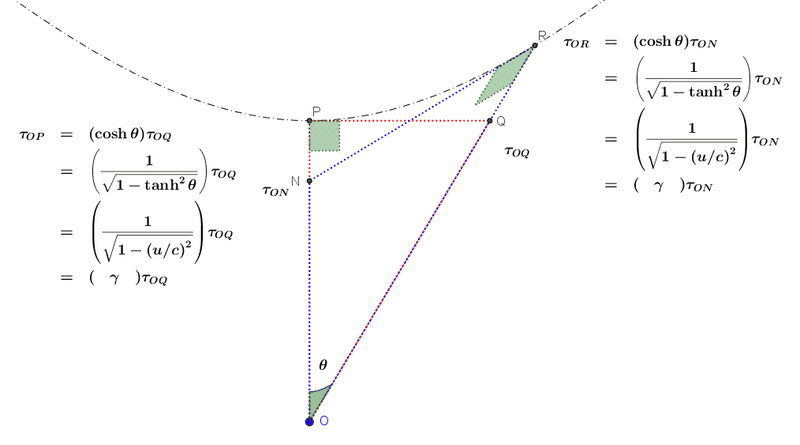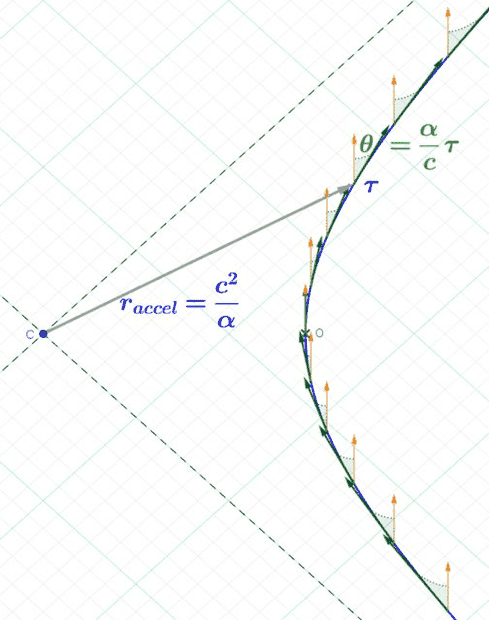From
https://physics.stackexchange.com/questions/667907/lorentz-transform-moving-length-to-moving-length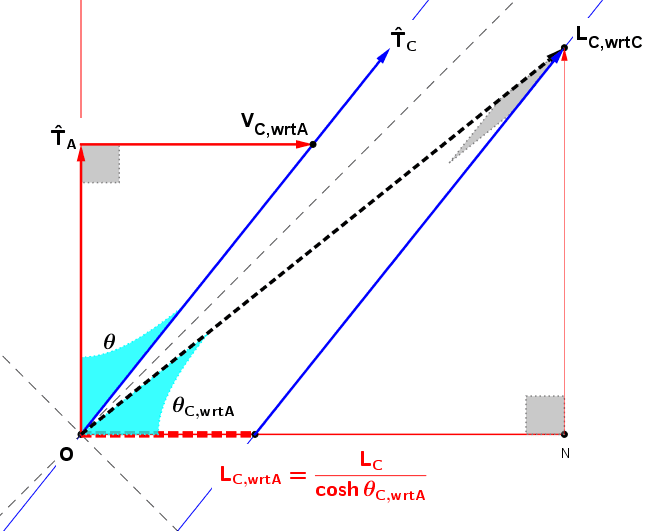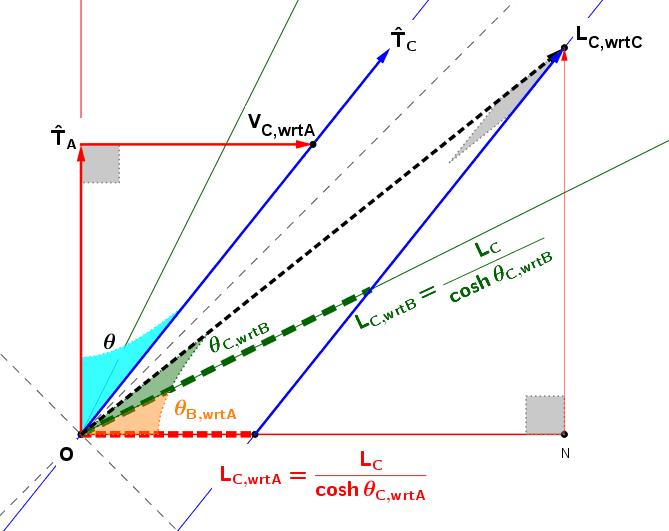In my opinion, since we teach students to use trigonometry to solve free-body diagrams,
we can leverage that experience in trying to teach relativity via hyperbolic-trigonometry
(on the way to possibly having them work with vectors and tensors and use invariant thinking).
There has to be something to show the "geometry" encoded by the tensor-algebra.

As an example of the power of trigonometry, look at my answer to this question:
https://physics.stackexchange.com/q...-out-of-knowledge-kinetic-energy-in-lab-frame
The question asks for a derivation of
##|\vec{p}^{\text{com}}_{\pi}|=\frac{M_{p}\sqrt{T^{L}_{\pi}(T^{L}_{\pi}+2M_{\pi})}}{\sqrt{(M_{p}+M_{\pi})^{2}+2T^{L}_{\pi} M_{p}}}##
and wonders about whether it is dependent on the frame of reference.

as the altitude of a triangle CB in connection with the Law of Sines in Minkowski spacetime.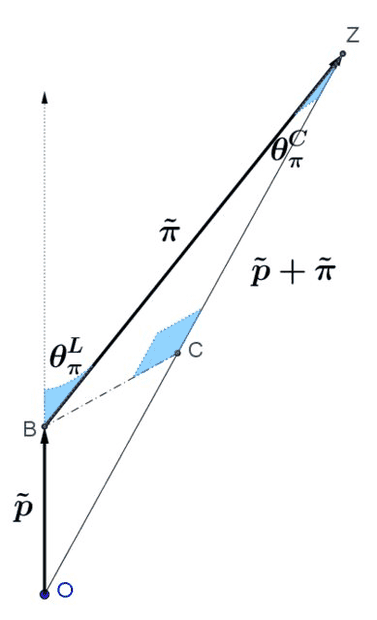Here is the muon problem solved trigonometrically (however, no angular arcs shown)
from https://physics.stackexchange.com/q...-distance-traveled-and-the-times-it-takes-why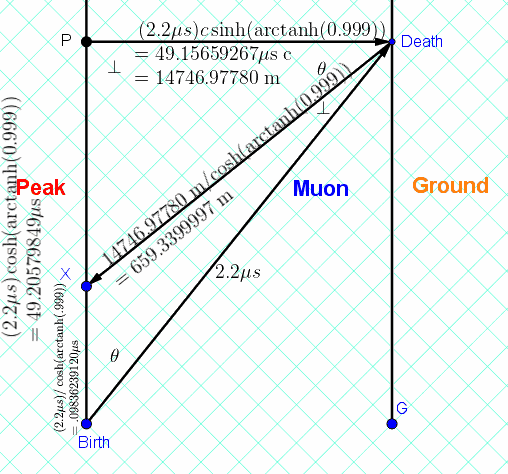To play around with the analogy,
try https://www.desmos.com/calculator/emqe6uyzha

Set ##E= -1##, then play around.
Move the slider slowly back to ##E= +1##.
See https://www.physicsforums.com/threads/why-is-time-orthogonal-to-space.969731/post-6159973 for a description of an earlier version of this.

Last edited:
•Kashmir and topsquark
So you defined rapidity ##\theta## as area that lies inside two lines that touch the unit hyperbola . If rapidity is ##\theta## then one of the intersection point of hyperbola with the line is ##(cosh(\theta), sinh(\theta)##.

Now getting back to our figure :

View attachment 314558
The ##\theta## here is rapidity?
Along the lines of what I replied to your comment in
https://physics.stackexchange.com/questions/728994/angle-in-a-spacetime-diagram/729066#729066

All of the events on the ##x'##-axis are on the line with slope ##ct=x \tanh\theta##.
Personally, I would reserve "rapidity" to refer to the angular-like measure between timelike lines. The indicated quantity between these spacelike-lines which are coplanar with the 4-velocities are numerically equal to the rapidity in Minkowski spacetime (but not in a Galilean spacetime).

Further, given two arbitrary spacelike lines in (3+1)-Minkowski spacetime,
a similarly-indicated measure of separation between those lines
need not be equal to the rapidity between the ##t##- and ##t'##-axes...
since those lines are not necessarily coplanar with the plane spanned by the time axes.

All of the events on the x′-axis are on the line with slope ct=xtanh⁡θ.
how does that imply all points on x' axis have the same hyperbolic angle ##\theta## given we use the sector definition of hyperbolic angle as shown by vanhees I am his green diagram above ?

how does that imply all points on x' axis have the same hyperbolic angle ##\theta## given we use the sector definition of hyperbolic angle as shown by vanhees I am his green diagram above ?
Any vector along that line cuts the unit hyperbola at same point... thus defining the same sector in the unit-hyperbola in this spacetime diagram. If you want the sector with the vector as one side of the sector, then divide the area of that sector by the square of the Minkowski-length of that vector.
Since there is no curvature in the plane, these sectors are similar to each other.
That's why the "angle" is independent of the radius.

(All of this is in analogy to what we do with "angle" in Euclidean space.)